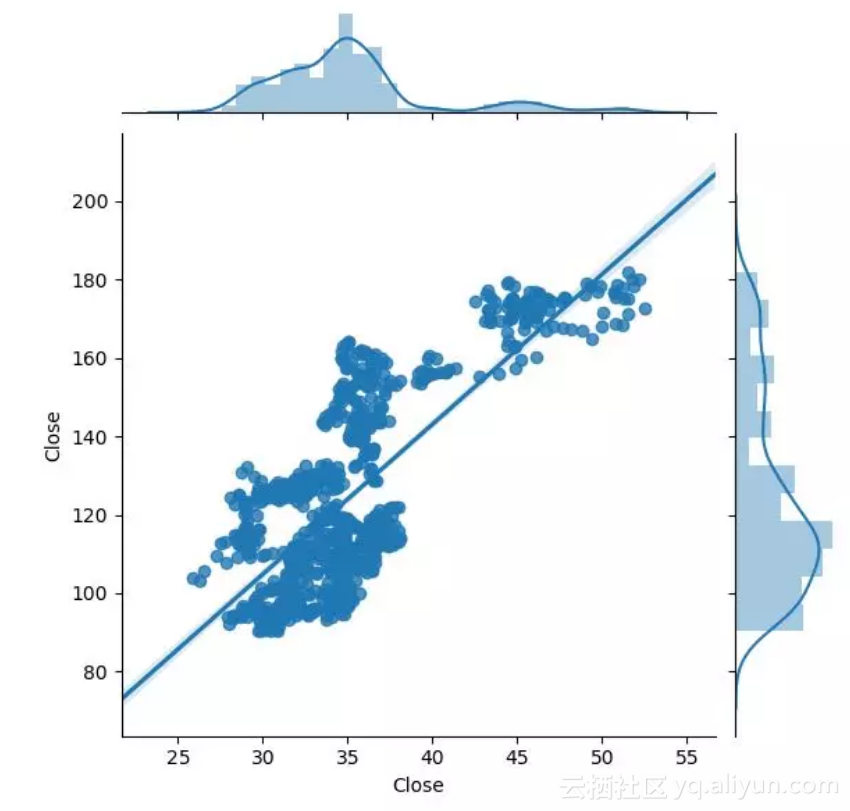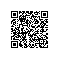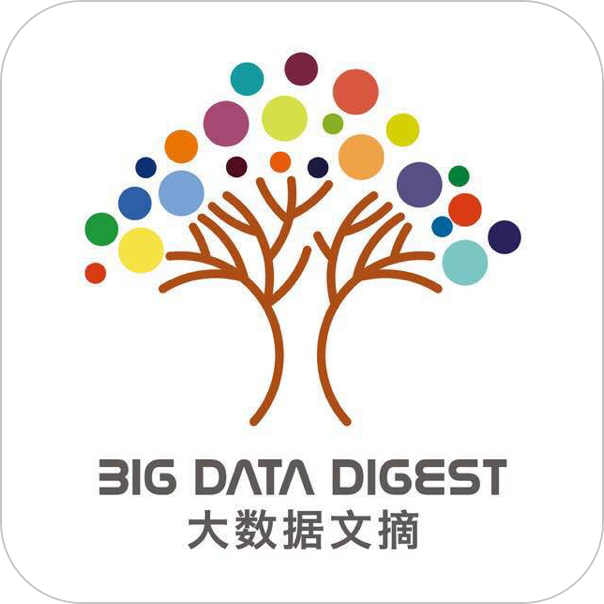# 驰骋股市！手把手教你如何用Python和数据科学赚钱？

Quantopian定义：

Apple苹果（AAPL）是一支很好的股票，因为目前为止（2018年9月）它已经是世界上价值最高的公司，不仅拥有相对稳定的股票价格，而且拥有足够多与品牌相关的体量、新闻和人气。

# create conda py35 since that's the newest version that works
conda create -n py35 python=3.5
conda install -c quantopian/label/ci -c quantopian zipline

import pandas as pd
import numpy as np
import patsy
pd.core.common.is_list_like = pd.api.types.is_list_like
import quandl
quandl.ApiConfig.api_key = "##############"

df = quandl.get("WIKI/" + 'AAPL', start_date="2014-01-01")

len(df)
df['Split Ratio'].value_counts()
df[df['Split Ratio'] == 7.0]

aapl_split = quandl.get("WIKI/" + 'AAPL', start_date="2014-06-10")

tickers = f500.Symbol.tolist()

# run ADF to determine unit root
import statsmodels.tsa.stattools as ts
print('Augmented Dickey Fuller:')
Augmented Dickey Fuller:
Test Statistic = -0.731194982176
p-value = 0.838503045276
Critical Values = {‘1%’: -3.4372231474483499, ‘5%’: -2.8645743628401763, ‘10%’: -2.5683856650361054}

Apple被认为是一个巨头技术品牌。假如我们能够计算与其他股票的强相关性会怎么样？

import matplotlib.pyplot as plt
import seaborn as sns
%matplotlib inline
MSFT = quandl.get("WIKI/" + 'MSFT', start_date="2014-06-10")
INTC = quandl.get("WIKI/" + 'INTC', start_date="2014-06-10")
TIF = quandl.get("WIKI/" + 'TIF', start_date="2014-06-10")

sns.jointplot(INTC.Close, aapl_split.Close, kind="reg");Intel vs. Apple我们还可以看看相关值数据( correlation value )：
np.corrcoef(INTC.Close, aapl_split.Close)

np.corrcoef(INTC.Close[:-7], aapl_split.Close[7:])

aapl_trends.tail()

API链接：
http://pandas.pydata.org/pandas-docs/stable/generated/pandas.DataFrame.resample.html
aapl_split_week = aapl_split.resample('W', convention='end').last()

# trend and price corr
np.corrcoef(aapl_trends['Apple: (Worldwide)'], aapl_split_week.Close)使用钉钉扫一扫加入圈子
+ 订阅